Eddie Resendiz

•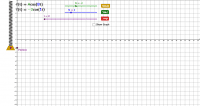Simple Harmonic Motion (Spring & Weight)

Activity

Eddie Resendiz

•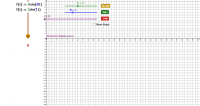Simple Harmonic Motion (Pendulum)

Activity

Eddie Resendiz

•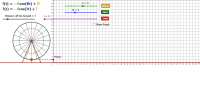Sinusoidal Functions(Ferris Wheel)

Activity

Eddie Resendiz

•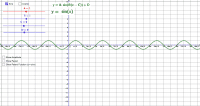Sine and Cosine Curves

Activity

Eddie Resendiz

•Multiplication Practice

Activity

Eddie Resendiz

•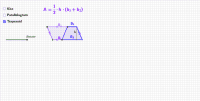Deriving Area Formulas

Activity

Eddie Resendiz

•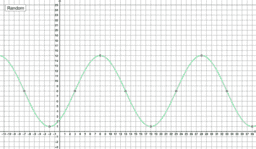Random Sinusoid Graph Generator

Activity

Eddie Resendiz

•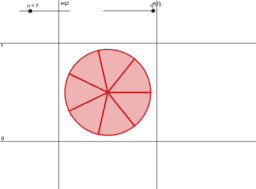Circle Fractions

Activity

Eddie Resendiz

•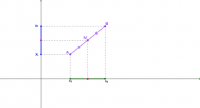Midpoint Visual

Activity

Eddie Resendiz

•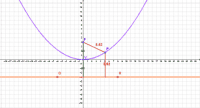Parabola Demo

Activity

Eddie Resendiz

•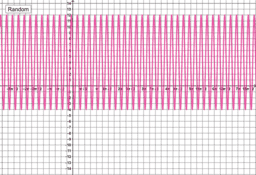Random Sine Curve Generator

Activity

Eddie Resendiz

•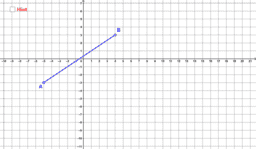Distance on a Coordinate Plane (with Hint)

Activity

Eddie Resendiz

•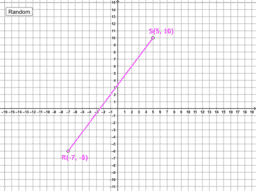Fractional Distance Generator

Activity

Eddie Resendiz

•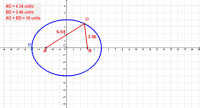Ellipse Demo

Activity

Eddie Resendiz

•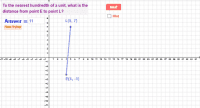Distance on a Coordinate Plane (Quiz)

Activity

Eddie Resendiz

•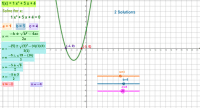Activity

Eddie Resendiz

•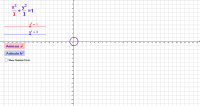Conics Equation Demo (Relationships between Conics)

Activity

Eddie Resendiz

•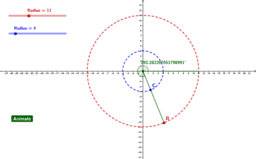Angular and Linear Speed

Activity

Eddie Resendiz

•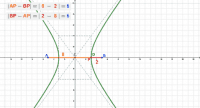Hyperbola Demo

Activity

Eddie Resendiz

•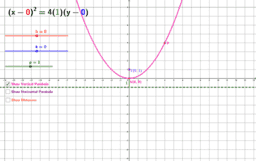Conics: Parabola Equation Demo

Activity

Eddie Resendiz

•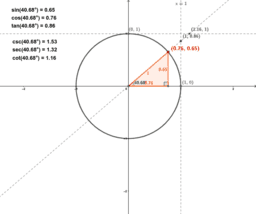Trigonmetric Functions Defined on a Unit Circle

Activity

Eddie Resendiz

•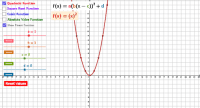Transformations of Parent Functions

Activity

Eddie Resendiz

•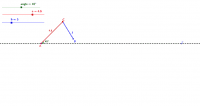SSA (Ambiguous Case)

Activity

Eddie Resendiz

•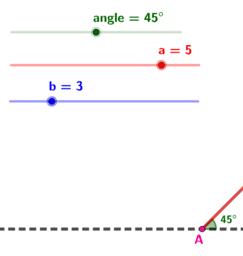Law of Sines - SSA (Ambiguous Case)

Activity

Eddie Resendiz